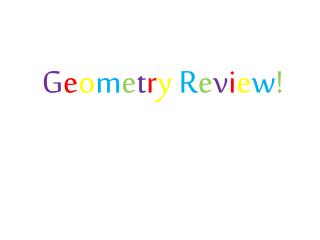DownloadDownload PresentationG e o m e t r y R e v i e w !

# G e o m e t r y R e v i e w !

Download Presentation## G e o m e t r y R e v i e w !

- - - - - - - - - - - - - - - - - - - - - - - - - - - E N D - - - - - - - - - - - - - - - - - - - - - - - - - - -
##### Presentation Transcript

1. GeometryReview!

4. 3. Draw and label each shape with it’s name, sides and angles. For example: 1 1 2 Square Sides: 4 Angles: 4 3 2 4 3 4 Trapezoid Pentagon Hexagon Triangle

5. 4.

6. 5.

7. Word Box: Thirds whole halves fourths 6. This circle shows: _____________ Write it as a fraction. 7. This circle shows: _____________ Write it as a fraction. 9. This circle shows: _____________ Write it as a fraction. 8. This circle shows: _____________ Write it as a fraction.

8. 10.

9. 11. Draw a shape with 6 angles!

10. Partner Activity! Create your task assigned to you on post-it paper. At the end of class or on Wednesday, each of you will present your paper to the rest of the class as a final review!

11. Shyanne and Evan I CAN IDENTIFY THE PROPERTIES OF A CUBE! Draw a LARGE cube in pencil. Circle all the vertices in Red Outline the edges in Green Write the amount of Faces, Vertices and Edges of a cube!

12. Eli and Kristen I can identify fractional parts. 2 Halves 1 whole 1 1 1 2 1 2 1 4 1 4 1 3 1 3 1 4 1 4 1 3 4 Fourths 3 Thirds

13. Isaac and Faith I can identify fractional parts. 2 Halves 1 whole 1 1 1 2 1 2 1 4 1 4 1 3 1 3 1 3 1 4 1 4 4 Fourths 3 Thirds

14. Hannah and Kacey I can identify a fraction. 1 4 Numerator – Part of the whole Denominator– The total amount in the whole Both of these objects show that one fourth is shaded in.

15. Sam and Ashley I can identify equal parts! Fractions must be cut into equal parts. Cuts made can be vertical, horizontal or diagonal. Here are 3 shapes cut into equal parts. Here are 3 shapes cut into unequal parts.

16. Casey and Kaela On your post – it, recreate our shape walk we made in our journals. Divide your paper into equal sections and place one shape in each! Make it big and colorful!! I CAN IDENTIFY 2D SHAPE PROPERTIES.

17. Daulton and Cheyenne I can make a whole! 1 1 1113 3 + 3 + 3 = 3 = 1 112 2 + 2 = 2 = 1 11114 4 + 4 + 4 + 4 = 4 = 1

18. Hope and Tyler I CAN IDENTIFY COLUMNS AND ROWS. I CAN FIND THE AREA OF A PARTITIONED RECTANGLE. **Create a visual to show that: Columns are VERTICAL ( up and down) and Rows are HORIZONTAL ( side to side) **Draw and label the answers to these questions Draw a rectangle with 3 columns and 4 rows. What is the total area inside? Draw a rectangle with 5 columns and 3 rows. What is the total area inside? Draw a rectangle with 7 columns and 5 rows. What is the total area inside. **Write these STRATEGIES at the bottom of your poster: Count the amount of squares inside the shape Multiply the number of columns by the number of rows or vice versa